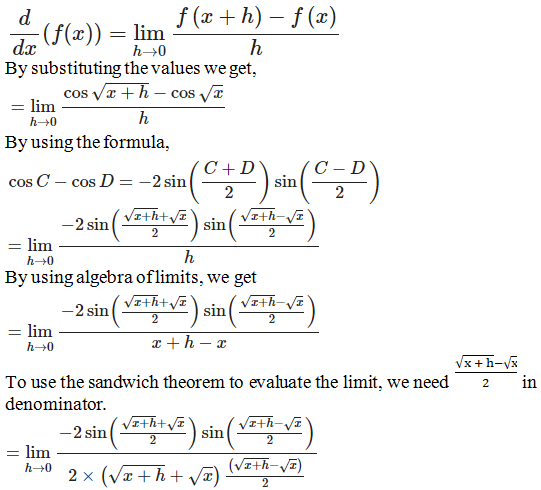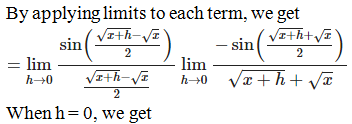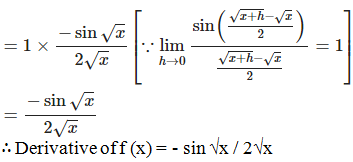# RD Sharma Solutions for Class 11 Chapter 30 - Derivatives Exercise 30.2

This exercise mainly discusses the concepts based on physical and geometrical interpretation of derivative at a point and differentiation from first principles. For better conceptual knowledge, students can refer to RD Sharma Class 11 Maths Solutions for good results in the board exams. The solutions here are developed by the expert tutors in order to help students secure good marks in the board exams. The RD Sharma Solutions are readily available in the pdf format which can be downloaded easily to start practising offline, for better results.

## Download the pdf of RD Sharma Solutions for Class 11 Maths Exercise 30.2 Chapter 30 – Derivatives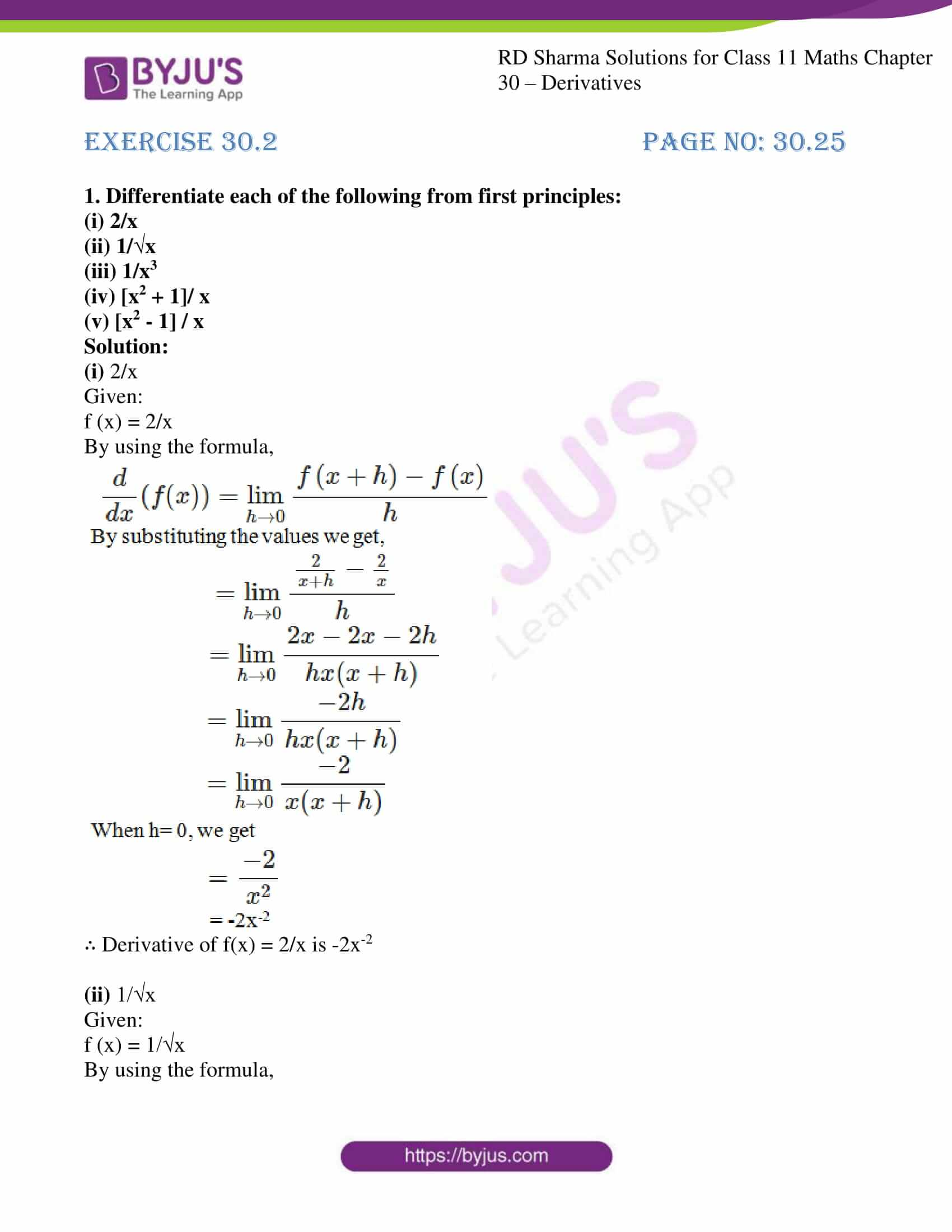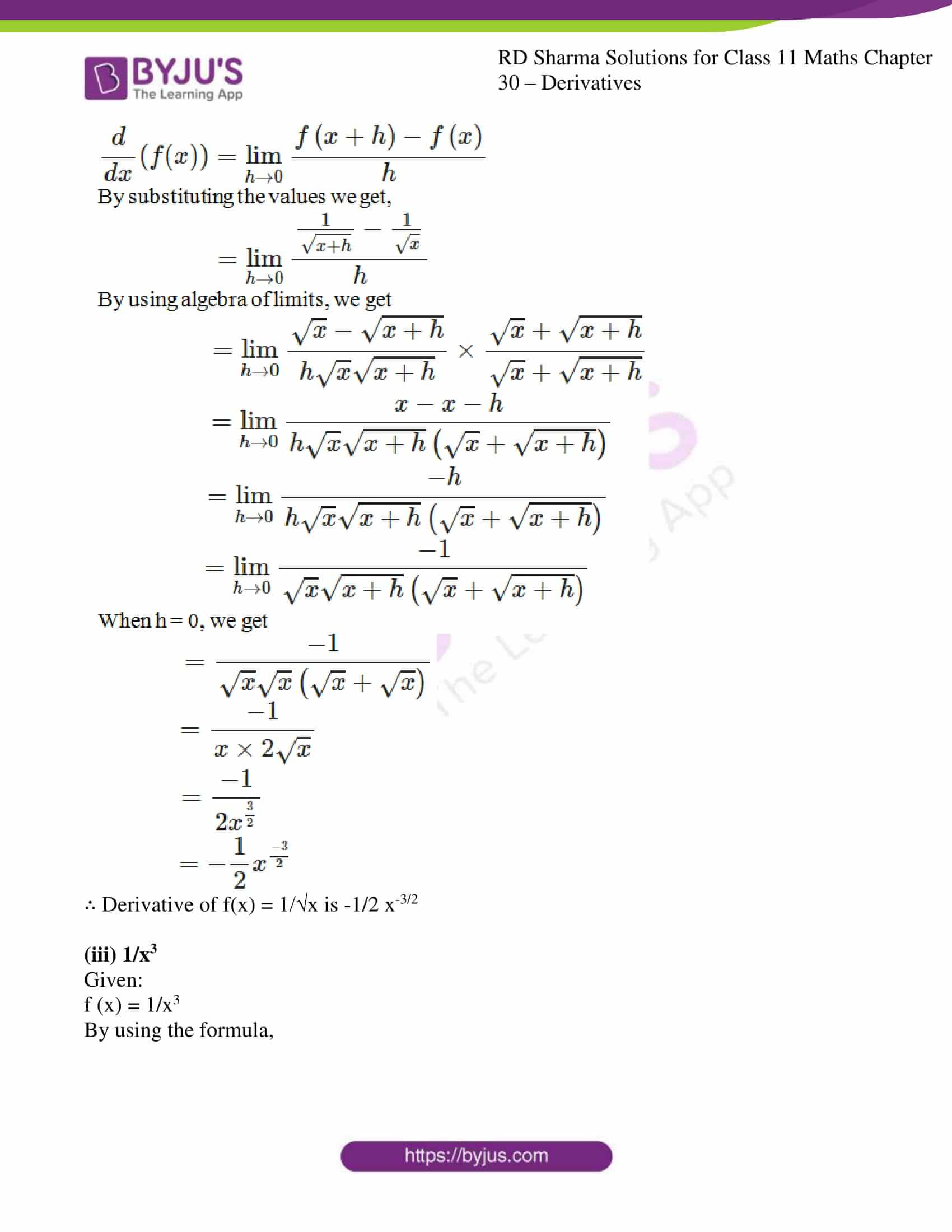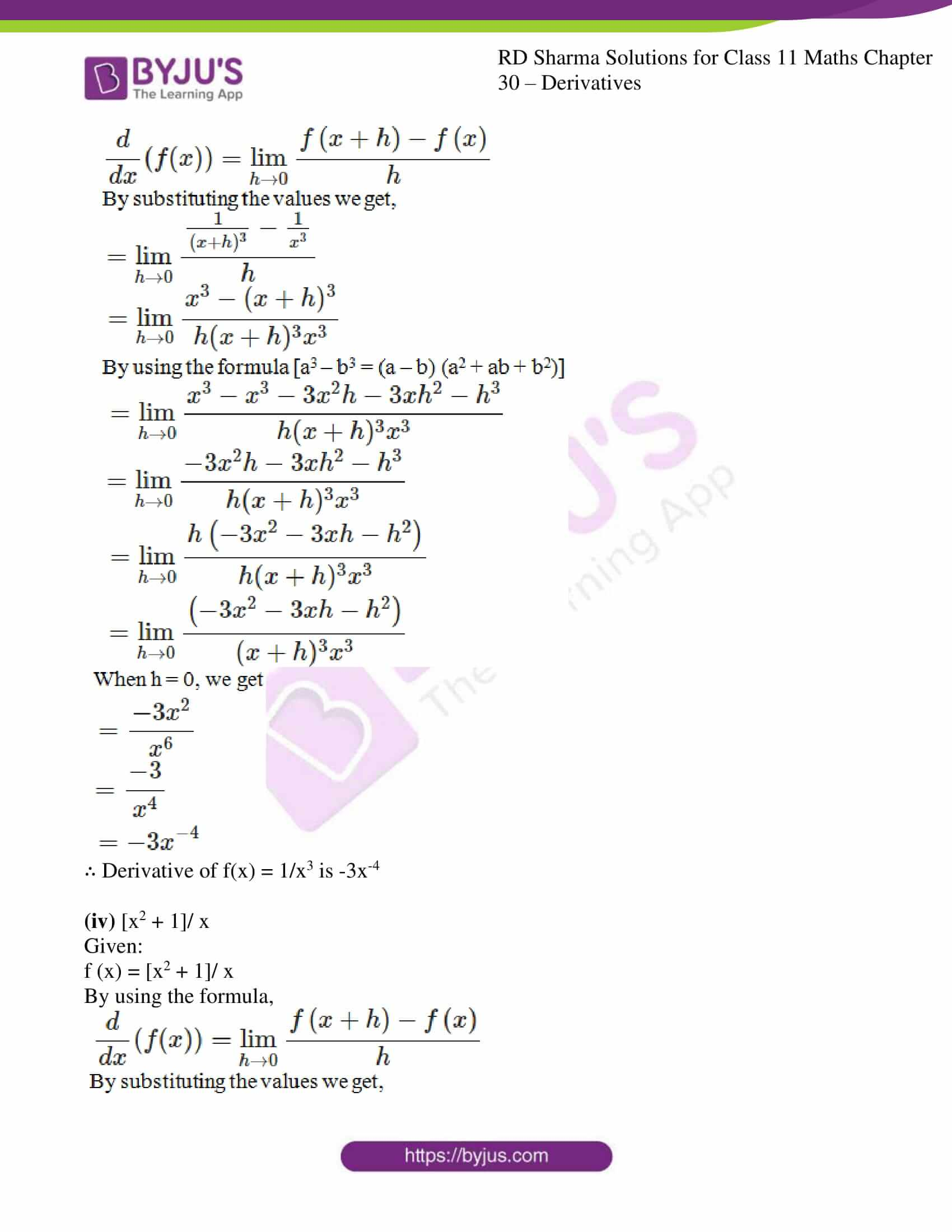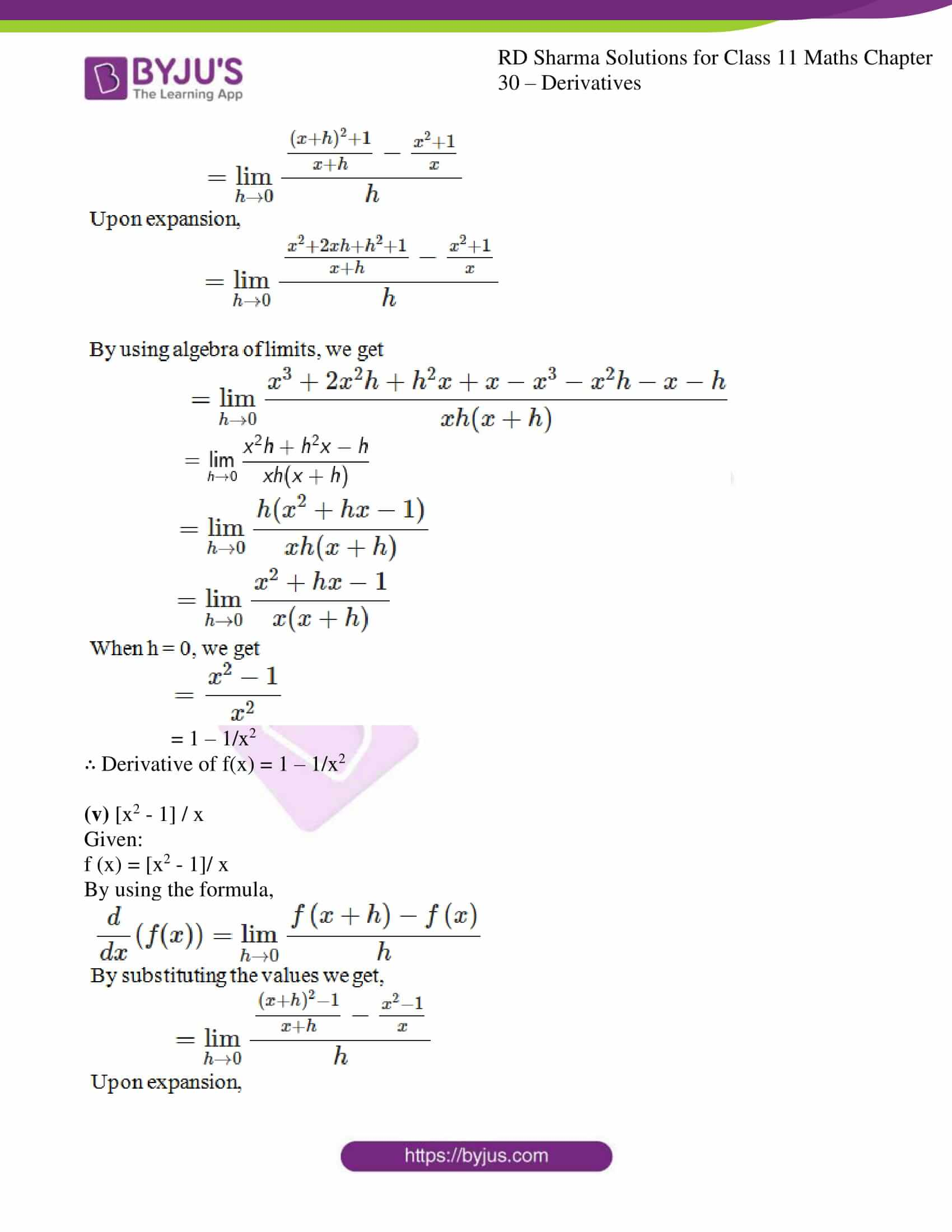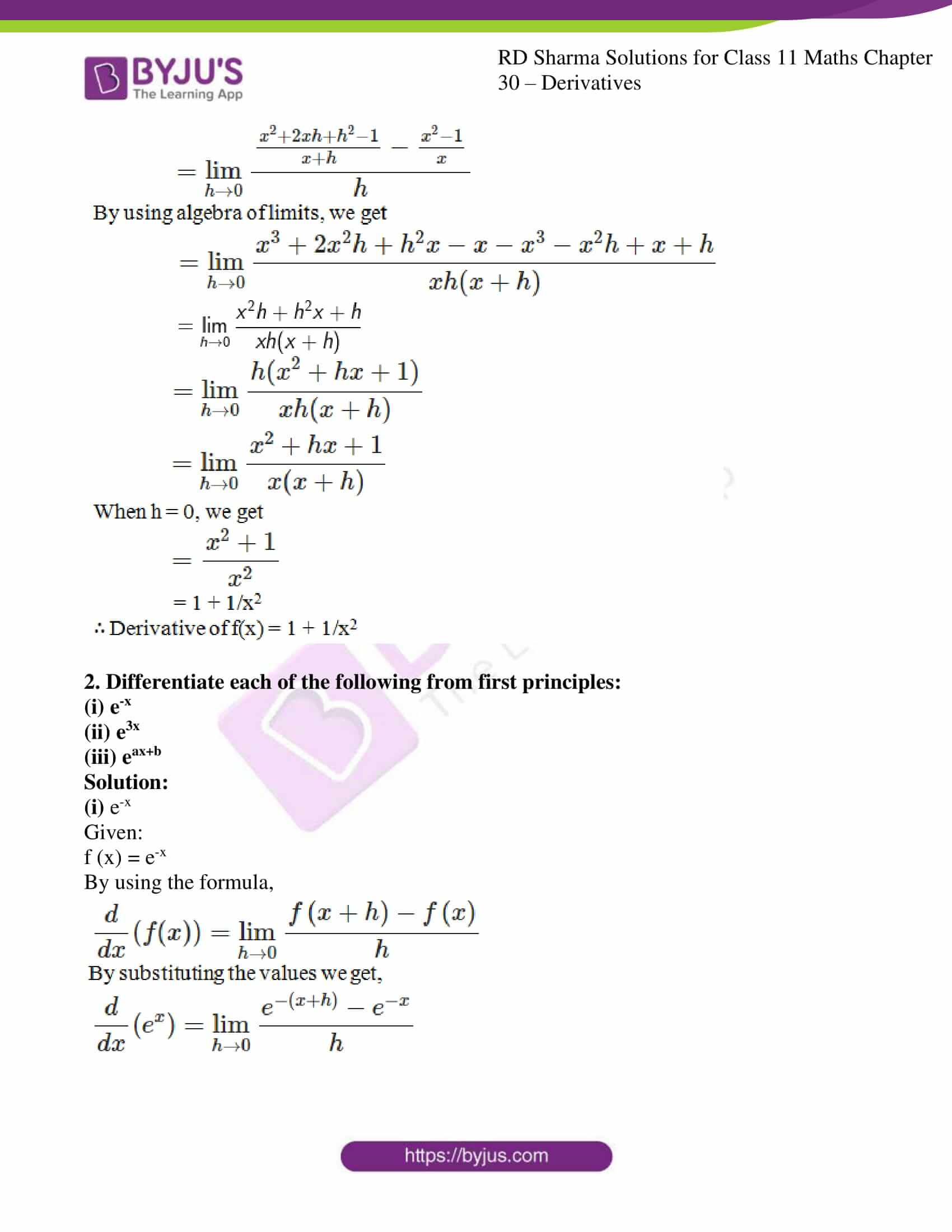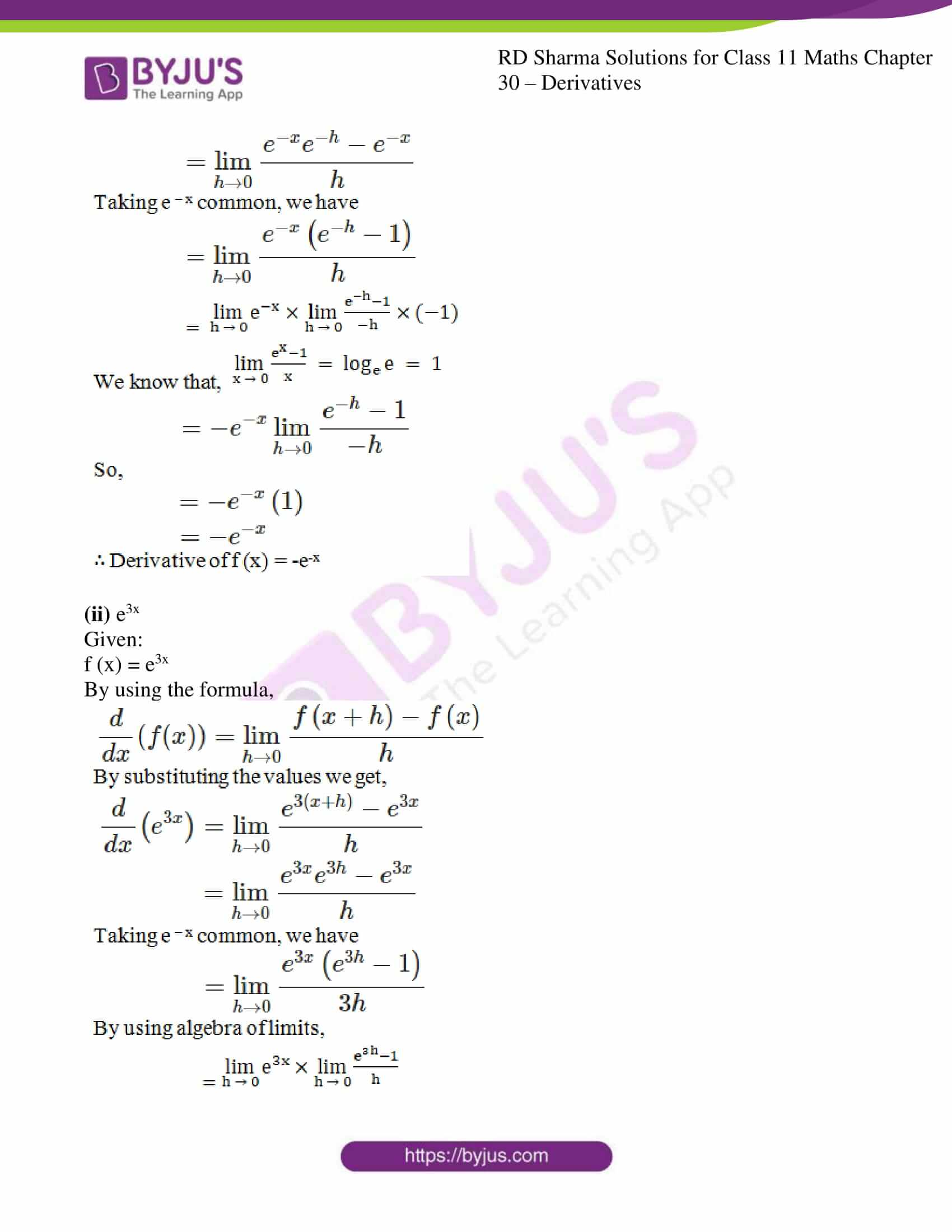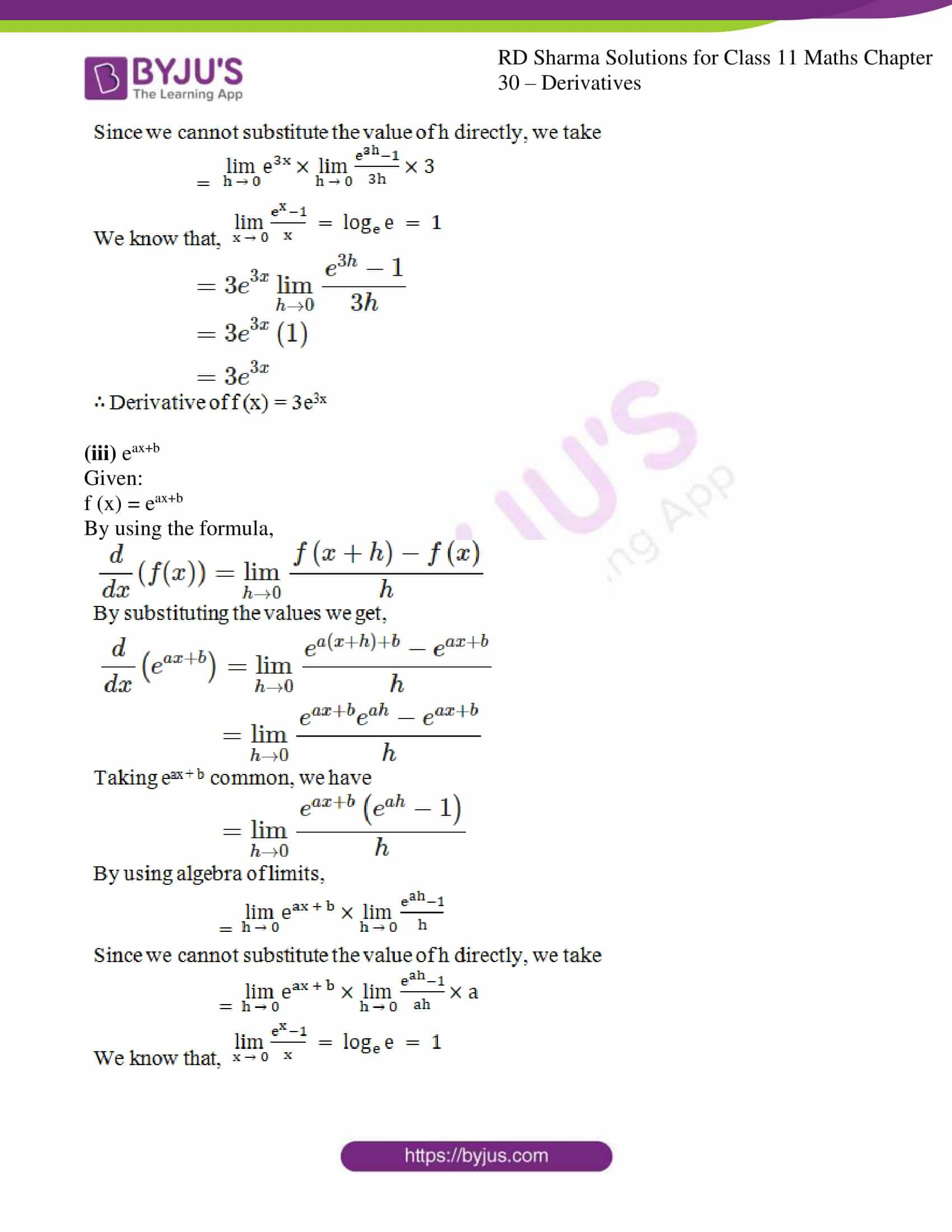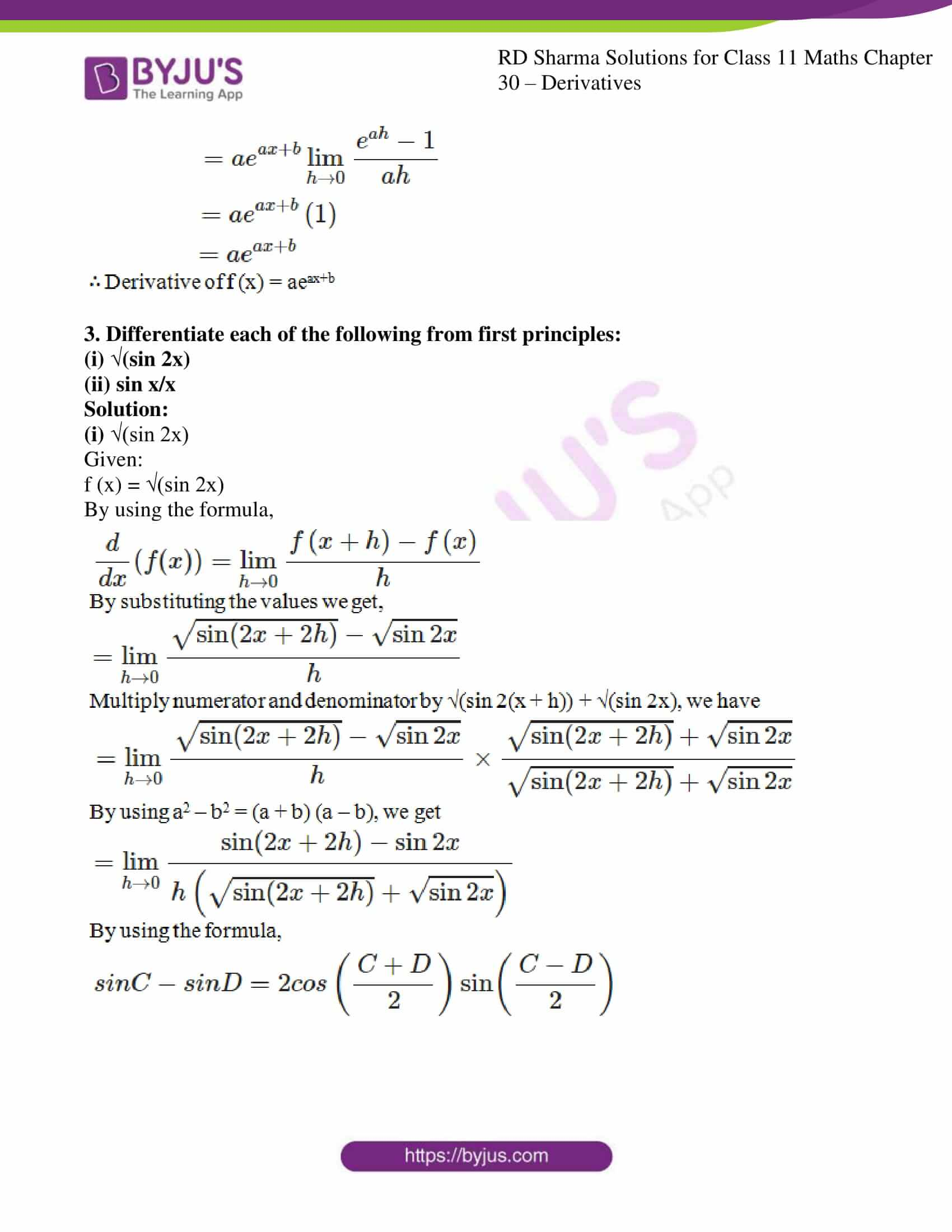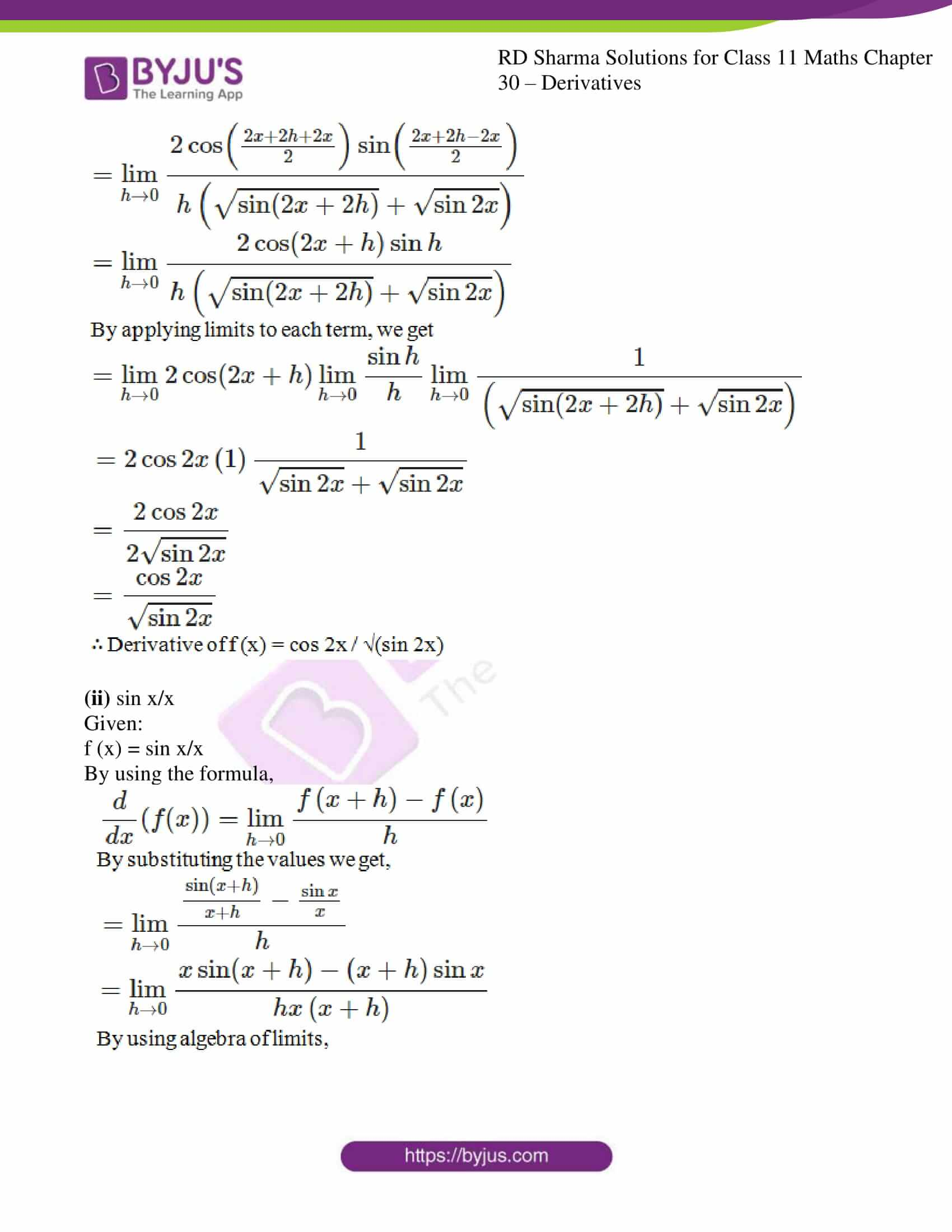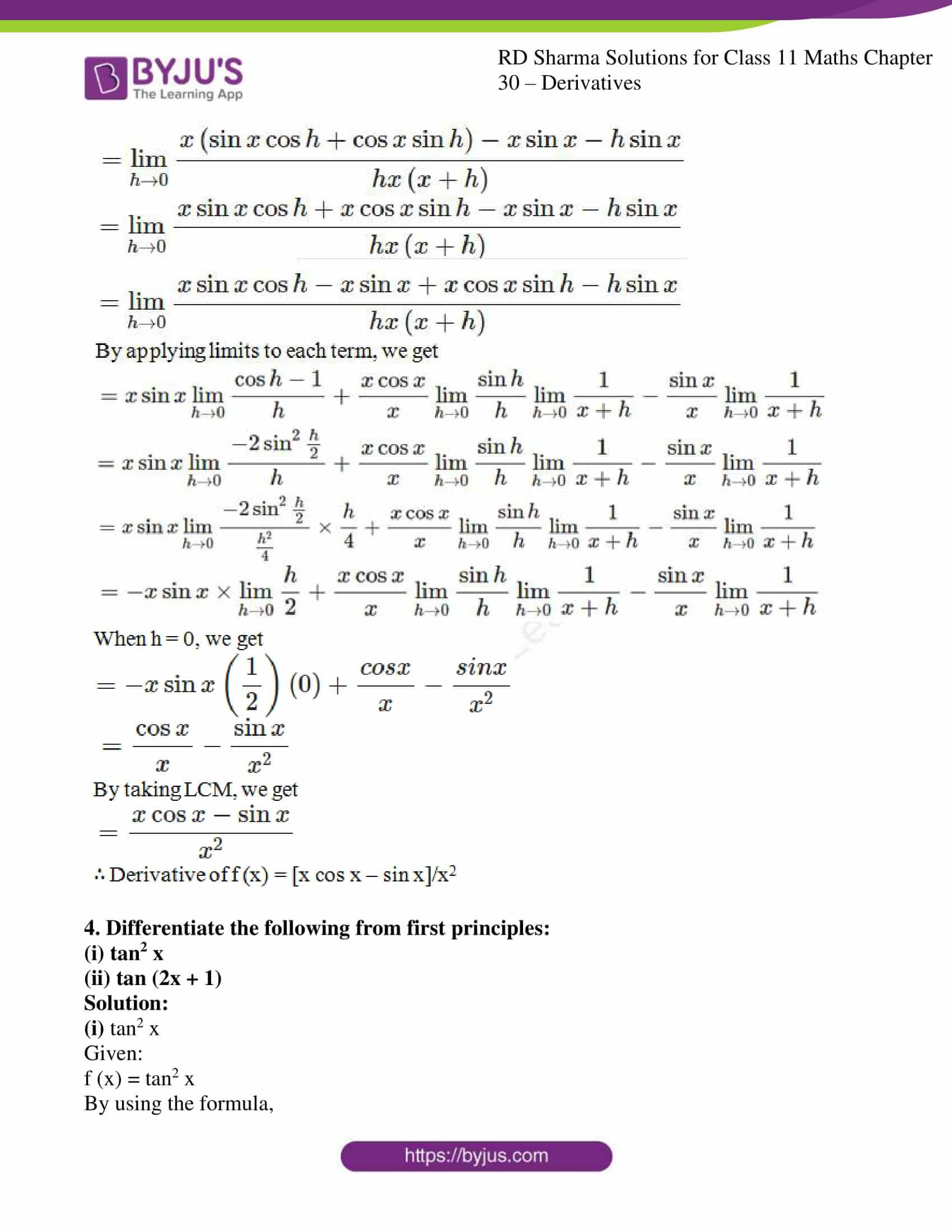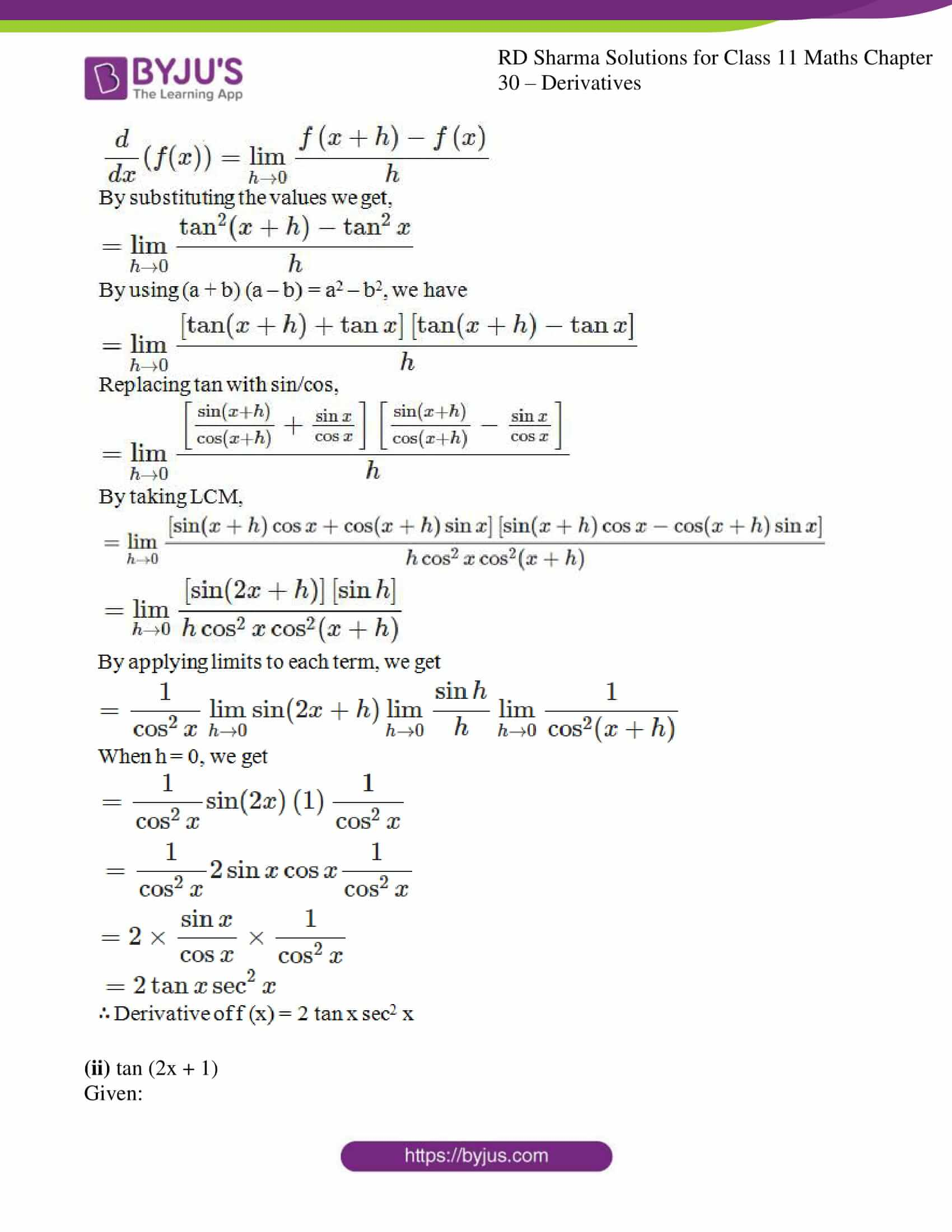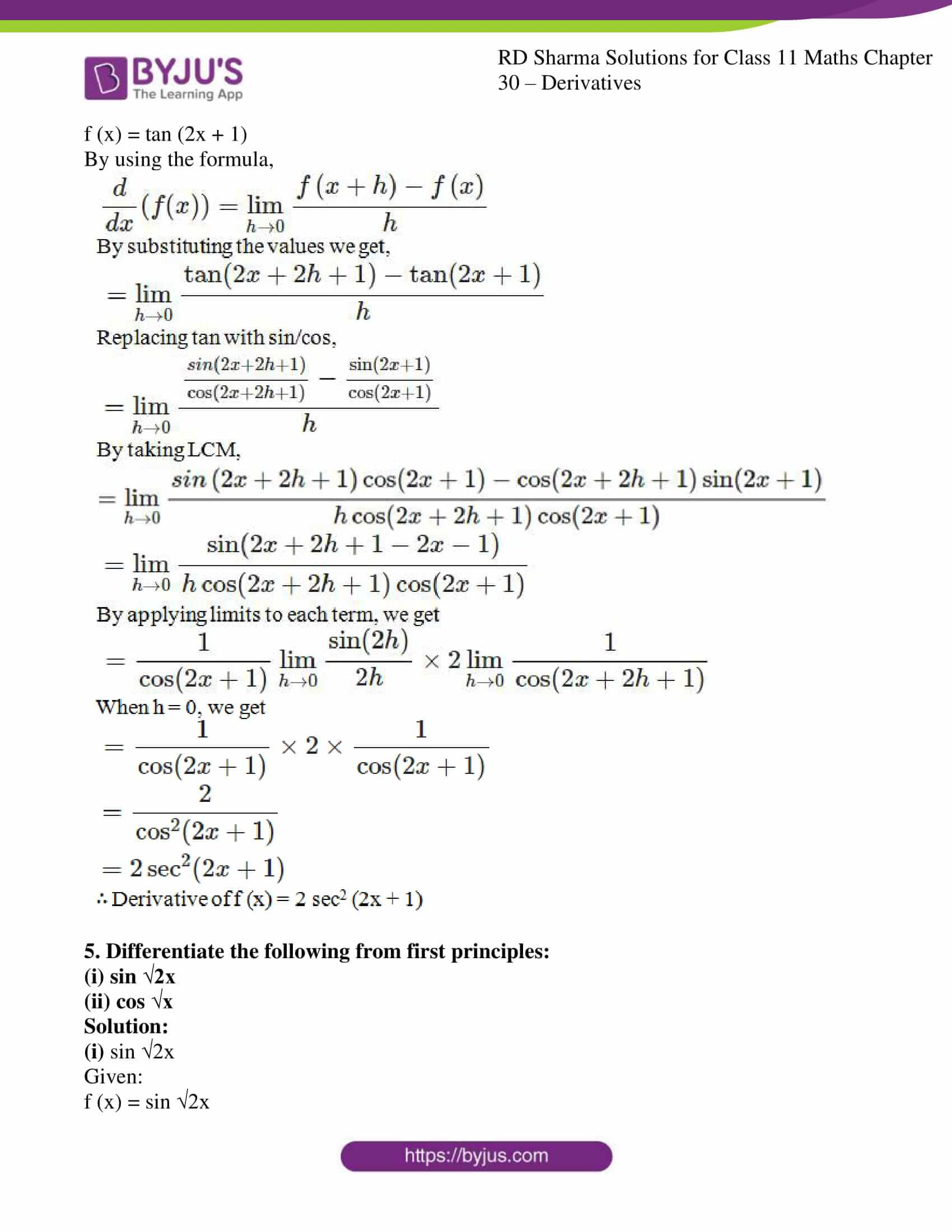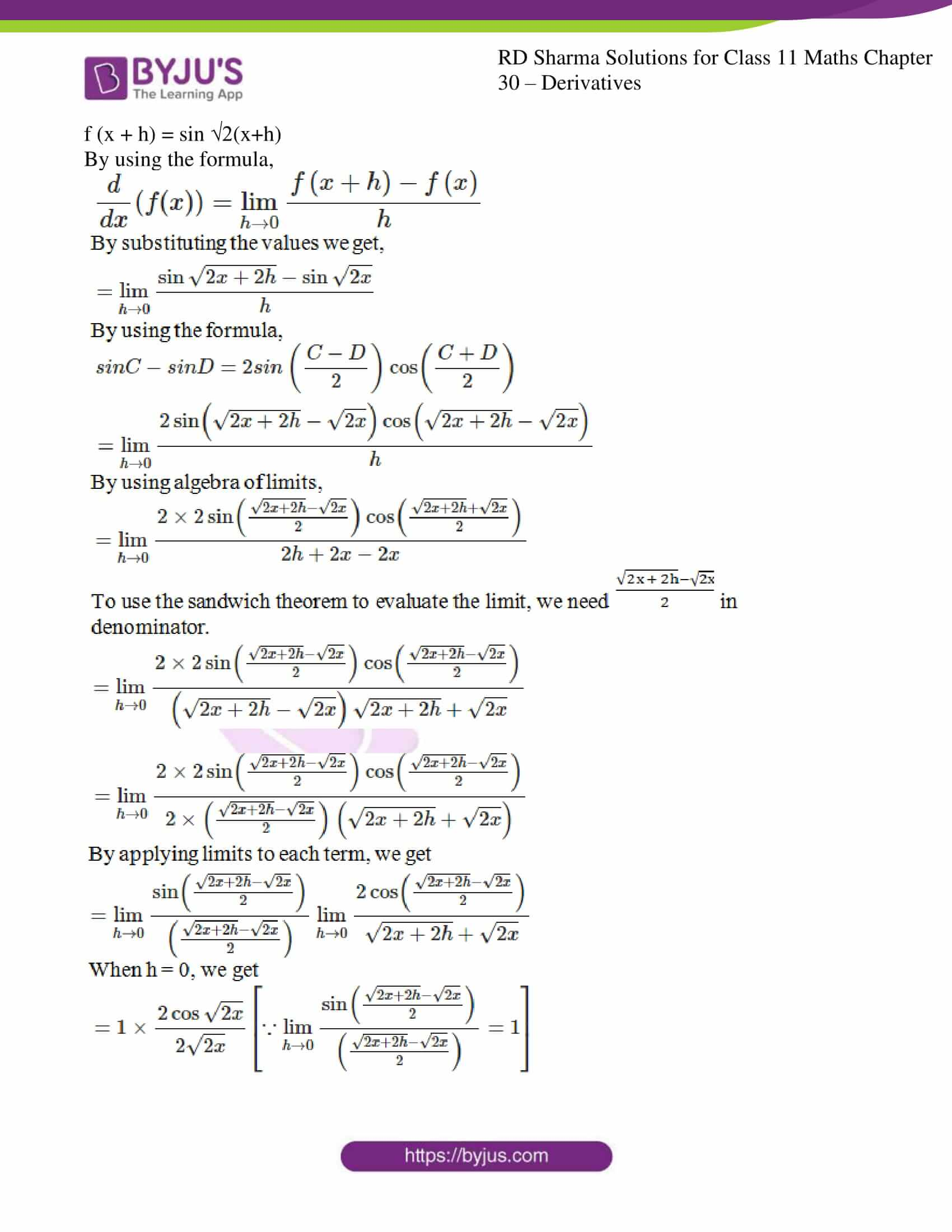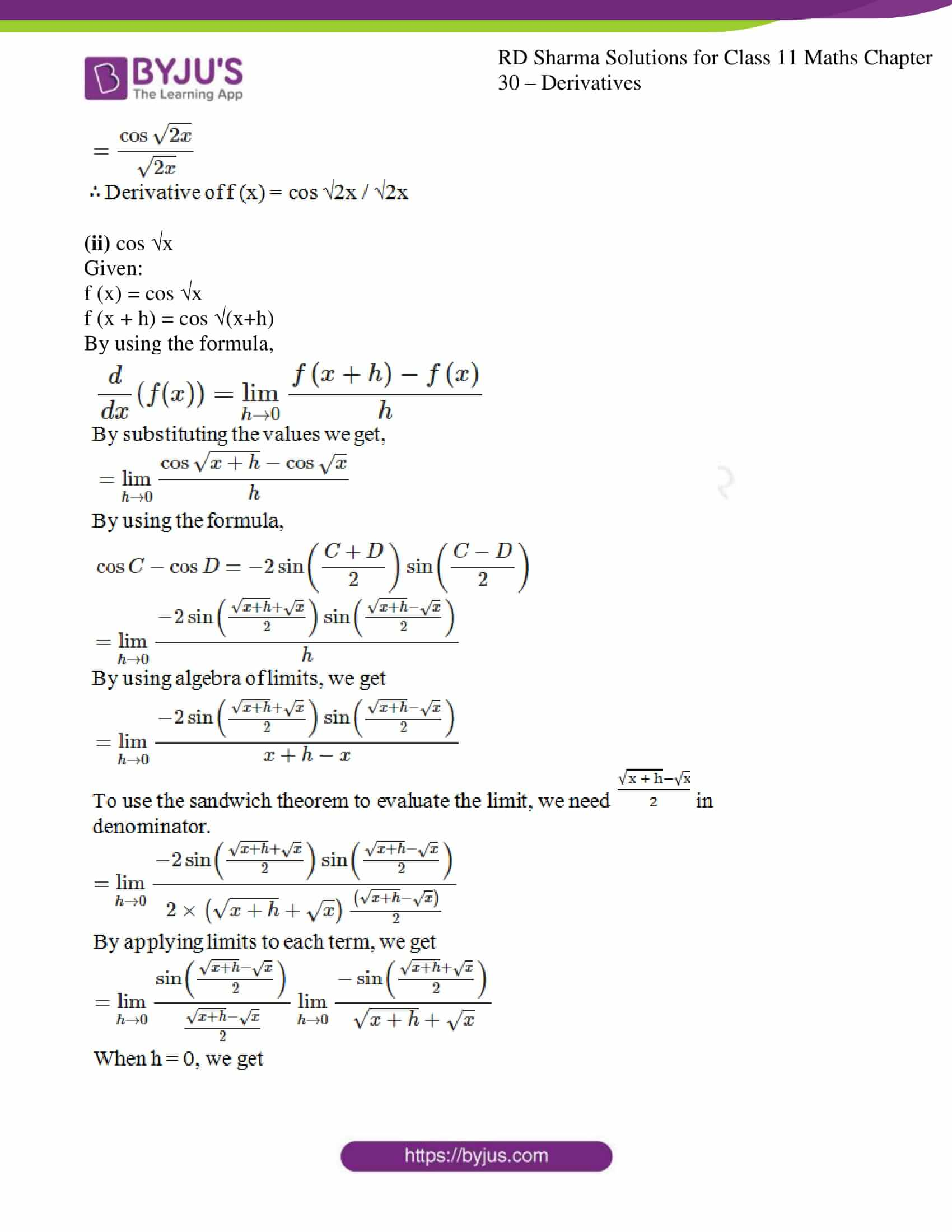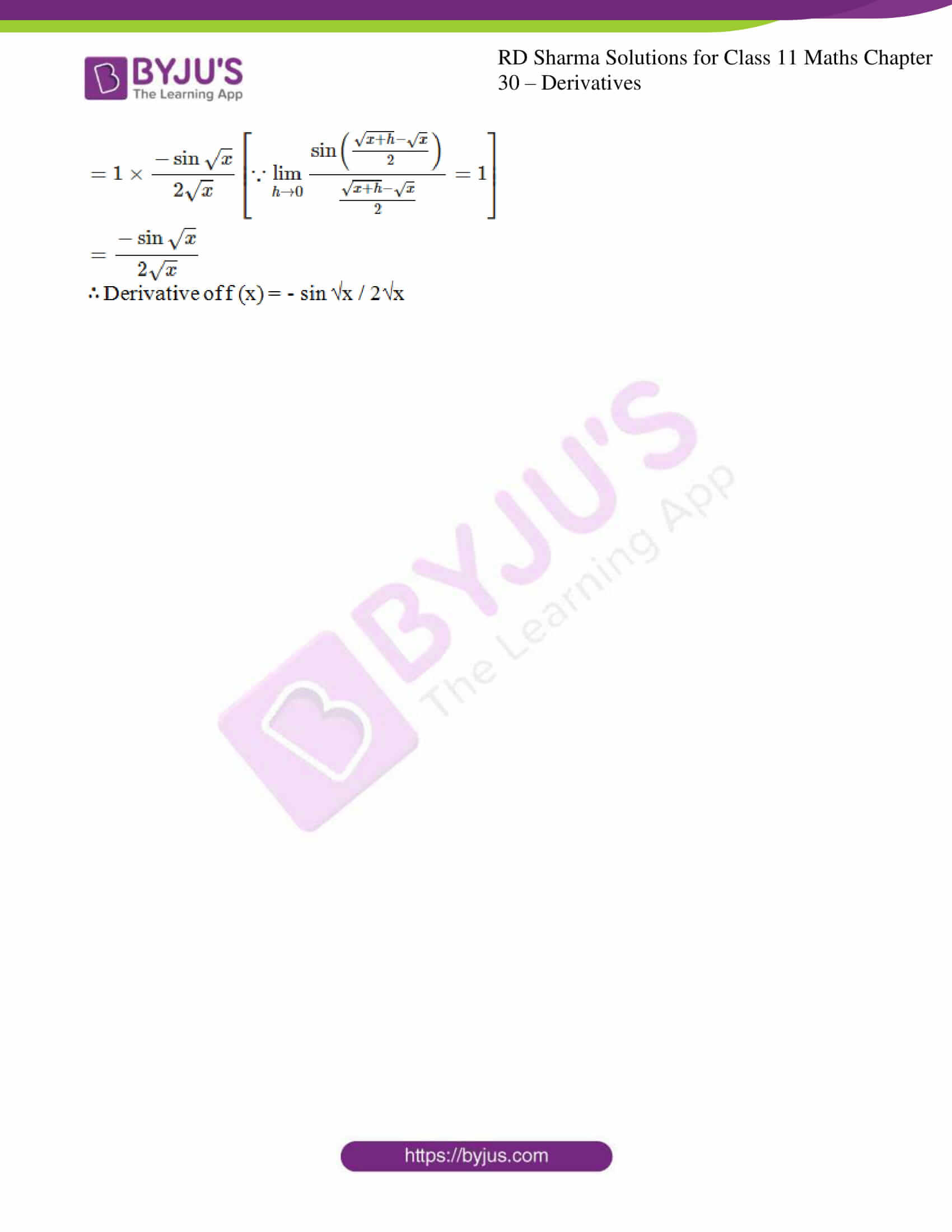### Access answers to RD Sharma Solutions for Class 11 Maths Exercise 30.2 Chapter 30 – Derivatives

#### EXERCISE 30.2 PAGE NO: 30.25

1. Differentiate each of the following from first principles:
(i) 2/x
(ii) 1/√x

(iii) 1/x3

(iv) [x2 + 1]/ x

(v) [x2 – 1] / x

Solution:

(i) 2/x

Given:

f (x) = 2/x

By using the formula,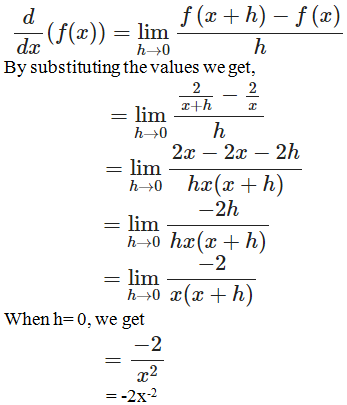∴ Derivative of f(x) = 2/x is -2x-2

(ii) 1/√x

Given:

f (x) = 1/√x

By using the formula,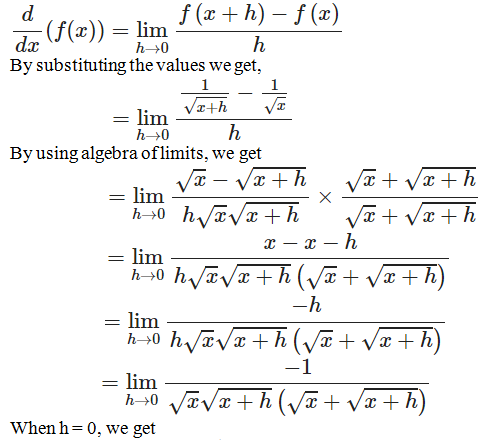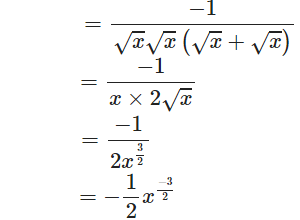∴ Derivative of f(x) = 1/√x is -1/2 x-3/2

(iii) 1/x3

Given:

f (x) = 1/x3

By using the formula,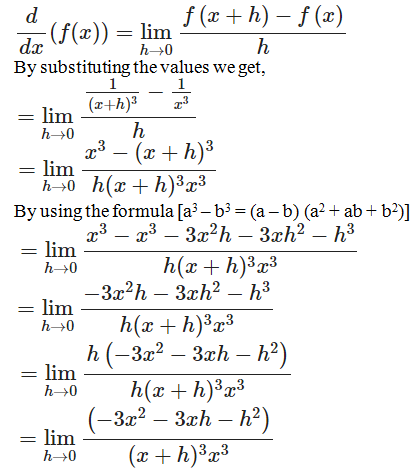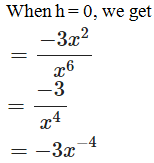∴ Derivative of f(x) = 1/x3 is -3x-4

(iv) [x2 + 1]/ x

Given:

f (x) = [x2 + 1]/ x

By using the formula,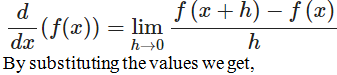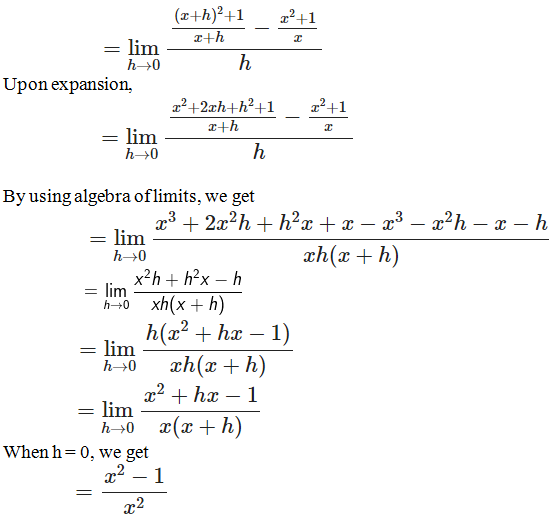= 1 – 1/x2

∴ Derivative of f(x) = 1 – 1/x2

(v) [x2 – 1] / x

Given:

f (x) = [x2 – 1]/ x

By using the formula,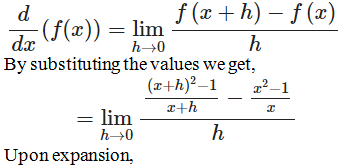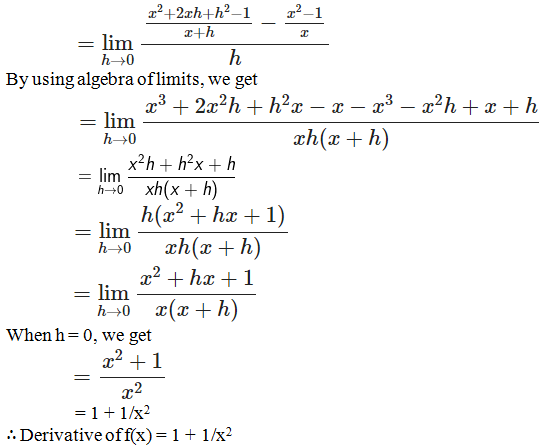2. Differentiate each of the following from first principles:

(i) e-x

(ii) e3x

(iii) eax+b

Solution:

(i) e-x

Given:

f (x) = e-x

By using the formula,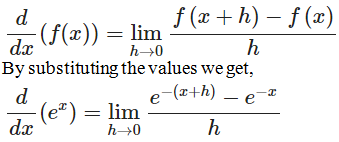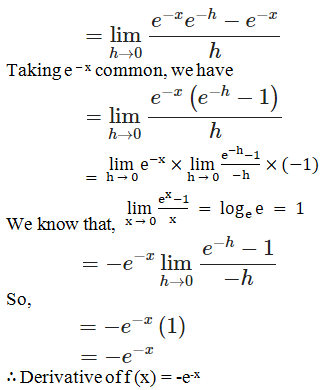(ii) e3x

Given:

f (x) = e3x

By using the formula,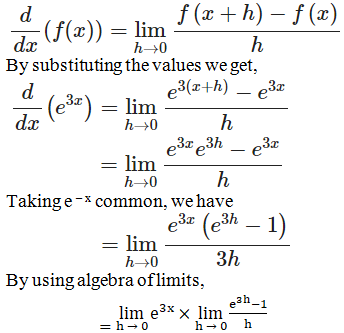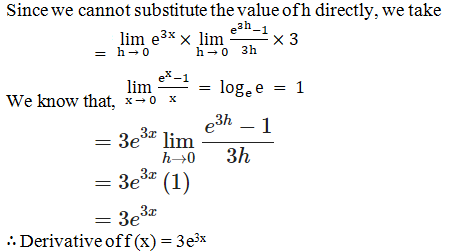(iii) eax+b

Given:

f (x) = eax+b

By using the formula,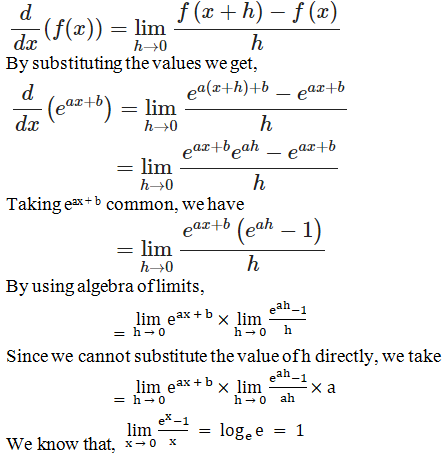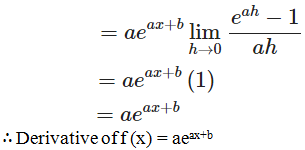3. Differentiate each of the following from first principles:

(i) √(sin 2x)

(ii) sin x/x

Solution:

(i) √(sin 2x)

Given:

f (x) = √(sin 2x)

By using the formula,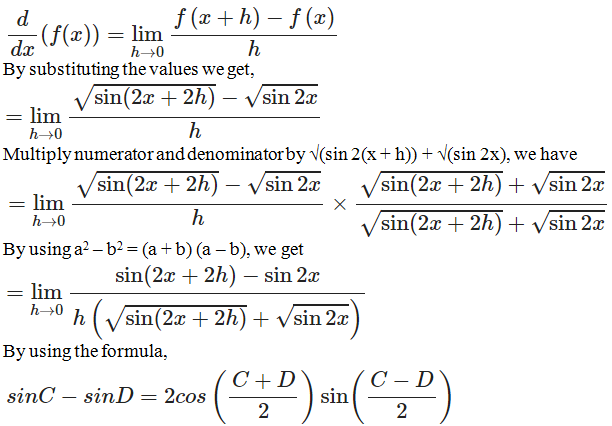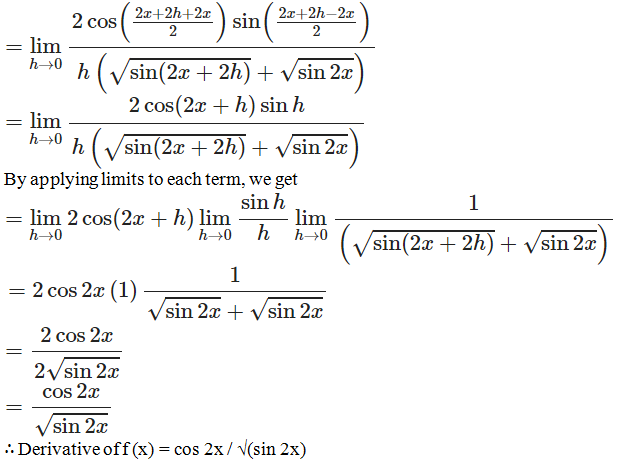(ii) sin x/x

Given:

f (x) = sin x/x

By using the formula,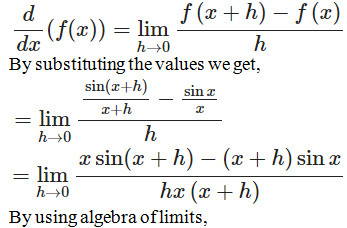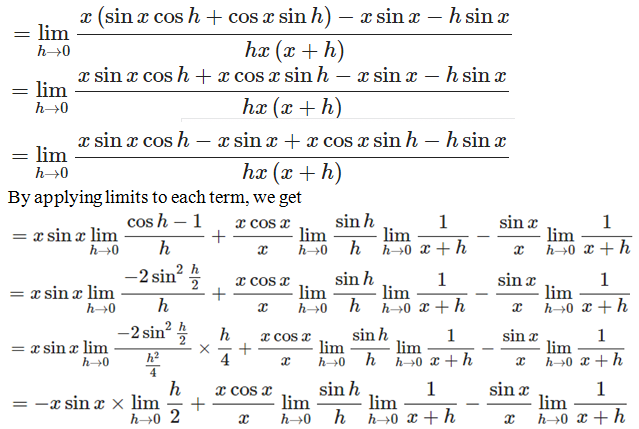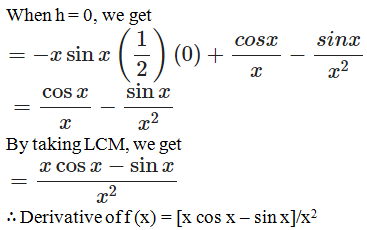4. Differentiate the following from first principles:

(i) tan2 x

(ii) tan (2x + 1)

Solution:

(i) tan2 x

Given:

f (x) = tan2 x

By using the formula,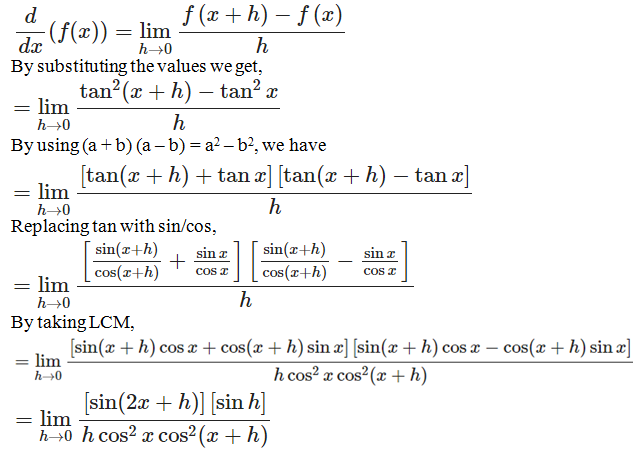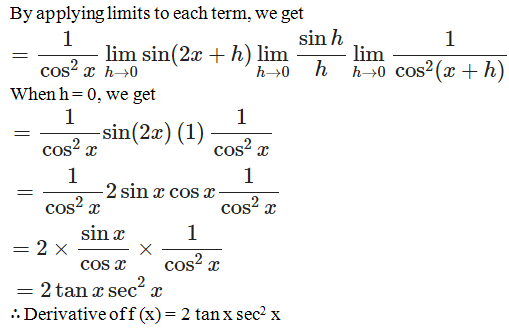(ii) tan (2x + 1)

Given:

f (x) = tan (2x + 1)

By using the formula,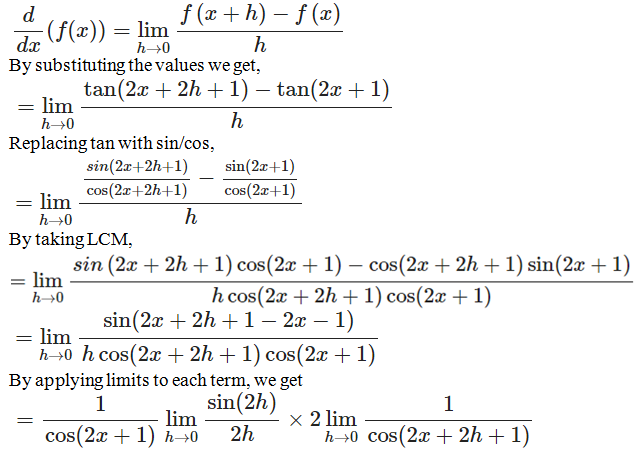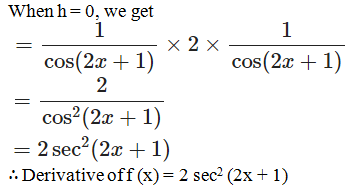5. Differentiate the following from first principles:

(i) sin √2x

(ii) cos √x

Solution:

(i) sin √2x

Given:

f (x) = sin √2x

f (x + h) = sin √2(x+h)

By using the formula,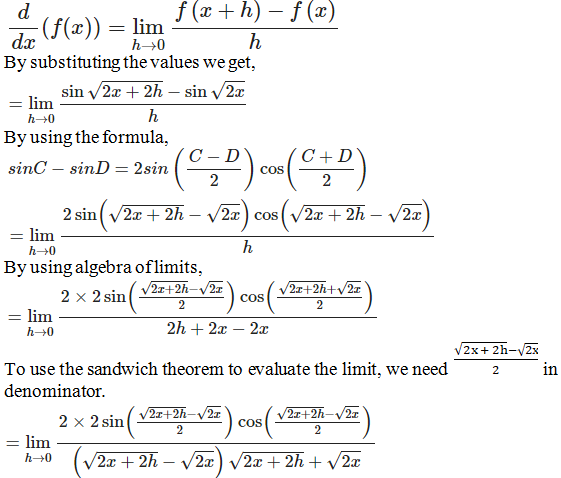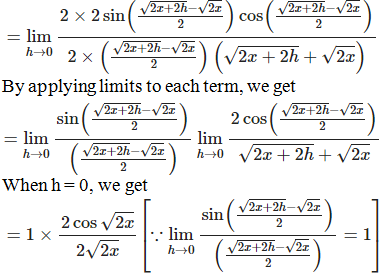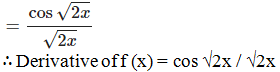(ii) cos √x

Given:

f (x) = cos √x

f (x + h) = cos √(x+h)

By using the formula,